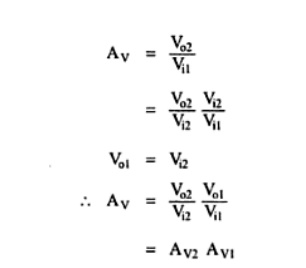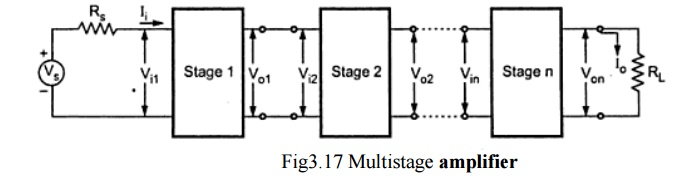Home | | Electronic Circuits I | Multistage Amplifiers

# Multistage Amplifiers

In practice, we need amplifier which can amplify a signal from a very weak source such as a microphone, to a level which is suitable for the operation of another transducer such as loudspeaker.

Multistage Amplifiers

In practice, we need amplifier which can amplify a signal from a very weak source such as a microphone, to a level which is suitable for the operation of another transducer such as loudspeaker. This is achieved by cascading number of amplifier stages, known as multistage amplifier

For faithful amplification amplifier should have desired voltage gain, current gain and it should match its input impedance with the source and output impedance with the load. Many times these primary requirements of the amplifier can not be achieved with single stage amplifier, because of the limitation of the transistor/FET parameters. In such situations more than one amplifier stages are cascaded such that input and output stages provide impedance matching requirements with some amplification and remaining middle stages provide most of the amplification.

We can say that,

When the amplification of a single stage amplifier is not sufficient, or,

When the input or output impedance is not of the correct magnitude, for a particular application two or more amplifier stages are connected, in cascade. Such amplifier, with two or more stages is also known as multistage amplifier.Vi1 is the input of the first stage and Vo2 is the output of second stage. So,Vo2/Vi1 is the overall voltage gain of two stage amplifier.Voltage gain :

The resultant voltage gain of the multistage amplifier is the product of voltage gains of the various stages.

Av = Avl Av2 ... Avn

Gain in Decibels

In many situations it is found very convenient to compare two powers on logarithmic scale rather than on a linear scale. The unit of this logarithmic scale is called decibel (abbreviated dB). The number N decibels by which a power P2 exceeds the power P1 is defined byDecibel, dB denotes power ratio. Negative values of number of dB means that the power P2 is less than the reference power P1 and positive value of number of dB means the power P2 is greater than the reference power P1.

For an amplifier, P1 may represent input power, and P2 may represent output power. Both can be given asWhere Ri and Ro are the input and output impedances of the amplifier respectively. Then,If the input and output impedances of the amplifier are equal i.e. Ri = Ro= R, then4. Gain of Multistage Amplifier in dB

The gain of a multistage amplifier can be easily calculated if the gain of the individual stages are known in dB, as shown below

20 log10 Av = 20 log10Avl + 20 log10Av2 +…      + 20 log10Avn

Thus, the overall voltage gain in dB of a multistage amplifier is the decibel voltage gains of the individual stages. It can be given as

AvdB = AvldB + Av2dB + ... + AvndB

Advantages of Representation of Gain in Decibels

Logarithmic scale is preferred over linear scale to represent voltage and power gains because of the following reasons :

·        In multistage amplifiers, it permits to add individual gains of the stages to calculate overall gain.

·        It allows us to denote, both very small as well as very large quantities of linear, scale by considerably small figures.

For example, voltage gain of 0.0000001 can be represented as -140 dB and voltage gain of 1,00,000 can be represented as 100 dB.

·        Many times output of the amplifier is fed to loudspeakers to produce sound which is received by the human ear. It is important to note that the ear responds to the sound intensities on a proportional or logarithmic scale rather than linear scale. Thus use of dB unit is more appropriate for representation of amplifier gains.

Study Material, Lecturing Notes, Assignment, Reference, Wiki description explanation, brief detail
Electronic Circuits : JFET and MOSFET Amplifiers : Multistage Amplifiers |